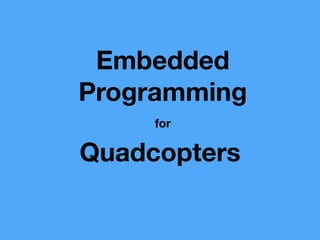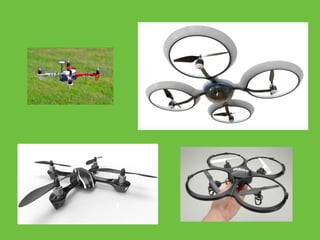Successfully reported this slideshow.

20

Share×
1 of 69
1 of 69

20

Share

A talk about how I designed, built and programmed by quadcopter from scratch. Details about reading from sensors and algorithm for motor outputs.

A talk about how I designed, built and programmed by quadcopter from scratch. Details about reading from sensors and algorithm for motor outputs.

## More Related Content

### Related Books

Free with a 14 day trial from Scribd

See all

### Related Audiobooks

Free with a 14 day trial from Scribd

See all

1. 1. Embedded Programming Quadcopters for
2. 2. I’m Ryan Boland Web Developer @ Tanooki Labs @bolandrm (github, twitter)
3. 3. 1. Components 2. Quadcopter physics 3. Sensor Inputs 4. Motor Outputs 5. Safety
4. 4. Frame
5. 5. Electronic Speed Controllers (ESCs) & Motors
6. 6. Lithium Polymer (LiPo) Battery
7. 7. Remote Control Transmitter + Receiver
8. 8. Flight Controller Microprocessor & Inertial measurement Unit (IMU)
9. 9. My Project - Custom Flight Controller Arduino Mega 2560 & Prototyping Shield 8-bit AVR 16 MHz clock 256K Flash 8K Ram Arduino Nano Clone 8-bit AVR 16 MHz clock 32K Flash 2K Ram Teensy 3.1 32-bit ARM 96 MHz clock 256K Flash 64K Ram \$5\$55 \$20
10. 10. My Project - Inertial Measurement Unit MPU6050 - 3 axis gyroscope, 3 axis accelerometer HMC5883L - 3 axis magnetometer BMP180 Barometer 3.3V or 5V GY-87 \$8
11. 11. Sourcing Components/Parts
12. 12. Conﬁguration - + vs X
13. 13. Orientation - Angles x axis == roll y axis == pitch z axis == yaw
14. 14. Maneuvering
15. 15. The Code
18. 18. Orientation (IMU) • rotational rates (x, y, z) (degrees per second) • angles (x, y) (degrees)
19. 19. IMU - Gyroscope measures rotational rate in °/sec
20. 20. IMU - Gyroscope average = -2.599 (°/s)
21. 21. Orientation (IMU) • rotational rates (x, y, z) (degrees per second) • angles (x, y) (degrees)
22. 22. Gyroscope - Angles Rotational Rate Duration Total Movement Quad Angle 0 0 0 0 ° 5 °/s 2 s 10 ° 10 ° -10 °/s 2 s -20 ° -10 ° -5 °/s 1 s -5 ° -15 °
23. 23. Gyroscope - Angles uint32_t gyro_last_update = micros(); void compute_gyro_angles() { mpu6050_read_gyro(&gyro_rates); rates.x = gyro_rates.x + GYRO_X_OFFSET; delta_t = (micros() - gyro_last_update) / 1000000; gyro_angles.x += rates.x * delta_t; gyro_last_update = micros(); }
24. 24. Gyroscope - Angles uint32_t gyro_last_update = micros(); void compute_gyro_angles() { mpu6050_read_gyro(&gyro_rates); rates.x = gyro_rates.x + GYRO_X_OFFSET; delta_t = (micros() - gyro_last_update) / 1000000; gyro_angles.x += rates.x * delta_t; gyro_last_update = micros(); }
25. 25. Gyroscope - Angles uint32_t gyro_last_update = micros(); void compute_gyro_angles() { mpu6050_read_gyro(&gyro_rates); rates.x = gyro_rates.x + GYRO_X_OFFSET; delta_t = (micros() - gyro_last_update) / 1000000; gyro_angles.x += rates.x * delta_t; gyro_last_update = micros(); }
26. 26. Gyroscope - Angles uint32_t gyro_last_update = micros(); void compute_gyro_angles() { mpu6050_read_gyro(&gyro_rates); rates.x = gyro_rates.x + GYRO_X_OFFSET; delta_t = (micros() - gyro_last_update) / 1000000; gyro_angles.x += rates.x * delta_t; gyro_last_update = micros(); }
27. 27. Gyroscope - Angles How is our estimation?
28. 28. Gyro Drift Occurs when gyroscope data changes between samples
29. 29. Orientation (IMU) • rotational rates (x, y, z) (degrees per second) • angles (x, y) (degrees) ?
30. 30. IMU - Accelerometer • measures acceleration in terms of g-force (g) • requires oﬀset calibration, similar to gyroscope data • z axis should be calibrated to 1G!
31. 31. IMU - Accelerometer http://www.freescale.com/ﬁles/sensors/doc/app_note/ AN3461.pdf (y, pitch) (x, roll) x = accel_filtered.x; y = accel_filtered.y; z = accel_filtered.z; accel_angles.x = atan2(y, z) * RAD_TO_DEG; accel_angles.y = atan2(-1 * x, sqrt(y*y + z*z)) * RAD_TO_DEG;
32. 32. IMU - Accelerometer http://www.freescale.com/ﬁles/sensors/doc/app_note/ AN3461.pdf (y, pitch) (x, roll) x = accel_filtered.x; y = accel_filtered.y; z = accel_filtered.z; accel_angles.x = atan2(y, z) * RAD_TO_DEG; accel_angles.y = atan2(-1 * x, sqrt(y*y + z*z)) * RAD_TO_DEG; (median ﬁlters)
33. 33. IMU - Accelerometer http://www.freescale.com/ﬁles/sensors/doc/app_note/ AN3461.pdf (y, pitch) (x, roll) x = accel_filtered.x; y = accel_filtered.y; z = accel_filtered.z; accel_angles.x = atan2(y, z) * RAD_TO_DEG; accel_angles.y = atan2(-1 * x, sqrt(y*y + z*z)) * RAD_TO_DEG;
34. 34. IMU - Accelerometer
35. 35. IMU - Accelerometer Susceptible to vibrations
36. 36. Combining Approaches Gyroscope - Good for short durations Accelerometer - Good for long durations Complementary Filter! #define GYRO_PART 0.995 #define ACC_PART 0.005 dt = <time since last update>; angles.x = GYRO_PART * (angles.x + (rates.x * dt)) + ACC_PART * accel_angles.x;
37. 37. Combining Approaches Gyroscope - Good for short durations Accelerometer - Good for long durations Complementary Filter! #define GYRO_PART 0.995 #define ACC_PART 0.005 dt = <time since last update>; angles.x = GYRO_PART * (angles.x + (rates.x * dt)) + ACC_PART * accel_angles.x;
38. 38. Combining Approaches Gyroscope - Good for short durations Accelerometer - Good for long durations Complementary Filter! #define GYRO_PART 0.995 #define ACC_PART 0.005 dt = <time since last update>; angles.x = GYRO_PART * (angles.x + (rates.x * dt)) + ACC_PART * accel_angles.x;
39. 39. Combining Approaches Gyroscope - Good for short durations Accelerometer - Good for long durations Complementary Filter! #define GYRO_PART 0.995 #define ACC_PART 0.005 dt = <time since last update>; angles.x = GYRO_PART * (angles.x + (rates.x * dt)) + ACC_PART * accel_angles.x;
40. 40. complementary ﬁlter vs previous approaches
41. 41. Orientation (IMU) • rotational rates (x, y, z) (degrees per second) • angles (x, y) (degrees)
43. 43. Remote Control http://rcarduino.blogspot.com/2012/01/how-to-read-rc- receiver-with.html Channel Function Min/Max Mapped Min/Max 1 Roll (1000μs, 2000μs) (-25, 25) 2 Pitch (1000μs, 2000μs) (-25, 25) 3 Throttle (1000μs, 2000μs) (1000, 2000) 4 Yaw (1000μs, 2000μs) (-50, 50)
45. 45. Controlling Motors (ESCs) Made to work with the remote control. Motor Max - 2000μs Motor Min - 1000μs
46. 46. Rate Mode
47. 47. 3 problems to correct Flight Controller Code
53. 53. The PI Controller (Proportional-Integral) • Calculates error based on diﬀerence between sensor reading and pilot command • Proportional term depends on present error • Integral term depends on accumulation of past errors
54. 54. The PI Controller (Proportional-Integral) #define KP 2.0 # ??? #define KI 2.0 # ??? float error = desired_pitch - current_pitch; proportional = KP * error; integral += KI * error * dt; output = proportional + integral;
55. 55. The PI Controller (Proportional-Integral) #define KP 2.0 # ??? #define KI 2.0 # ??? float error = desired_pitch - current_pitch; proportional = KP * error; integral += KI * error * dt; output = proportional + integral;
56. 56. The PI Controller (Proportional-Integral) #define KP 2.0 # ??? #define KI 2.0 # ??? float error = desired_pitch - current_pitch; proportional = KP * error; integral += KI * error * dt; output = proportional + integral;
57. 57. The PI Controller (Proportional-Integral) #define KP 2.0 # ??? #define KI 2.0 # ??? float error = desired_pitch - current_pitch; proportional = KP * error; integral += KI * error * dt; output = proportional + integral;